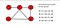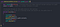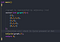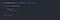# Hamiltonian Path and Cycle in the Graph using Backtracking.

Hamiltonian path and cycle are one of the important concepts in graph theory. In this blog, we will try to find out all the Hamiltonian paths and detect the hamiltonian cycle in the graph.

So firstly we will try to understand the hamiltonian path. Hamiltonian Path in a directed or undirected graph is a path that visits every vertex or edge only once. Consider the following graph and try to find out all hamiltonian paths in it.(a) Graph with all hamiltonian paths

Now our task is to print all the hamiltonian paths in this graph. How are we going to do that? Notice one thing that we always try to visit non-visited vertex. e.g 0 -> 1 -> 2 -> 3 -> 4 that means every vertex is getting visited once only.

But lets consider the path 3 -> 2 -> 1 -> 0 . Here vertex 4 remains non-visited. From vertex 0 there is no path to non-visited vertex 4. In order to visit vertex 4 , we have to visit 1 again and then visit 4 so the path will look like this 3 ->2 -> 1-> 0-> 1 -> 4, and according to the definition of the Hamiltonian path, it is an invalid path. I hope it is clear till now. Below is the c++ implementation for printing all the Paths.

In the hamiltonian paths function, we are going to explore paths starting from each vertex.Read the comments for understanding.

The output will print all the hamiltonian paths in a graph.

Hamiltonian Cycle is also a hamiltonian path with the edge between the last and starting vertex of the path.

The code for checking the hamiltonian cycle is almost similar. The only thing we have to do is to check Is there an edge between the last and first vertex of the path. Below is the C++ implementation for Hamiltonian Cycle.The graph is represented with an adjacency list

Notice we are calling the ‘ isCycleutil() ’ function with ‘0’ as a starting vertex, but we can start with any vertex as we are checking for the cycle. Because If there is a cycle in the graph, It can be detected from any vertex. You can start any vertex as well, starting vertex does not matter here.checkEdge() will check for an edge between the last and first vertex of a hamiltonian path

I hope this helped you to understand the Hamiltonian Cycle and Paths concept.

If you liked it Clap and comment down your feedback in the comment section.

Click on the Follow button for more amazing posts.

Thanks for reading it, Bye!

Undergraduate student at Pune University.

## More from Atharv Joshi

Undergraduate student at Pune University.